“6天+365天”一站式交易服务平台
•••••+
•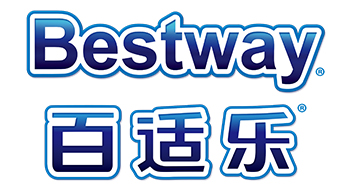+
•+
•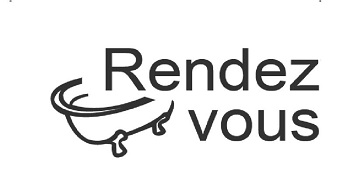+
•+
•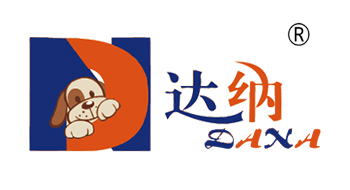+
•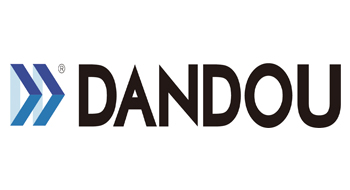+
•+
•+
•+
•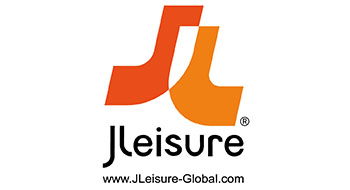+
•+
•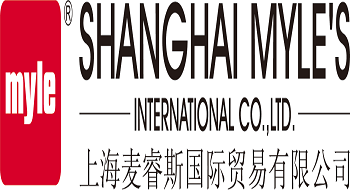+
•+
•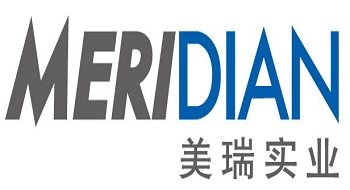+
•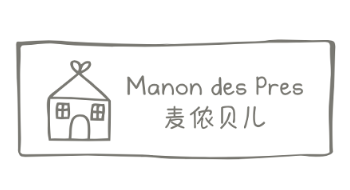+
•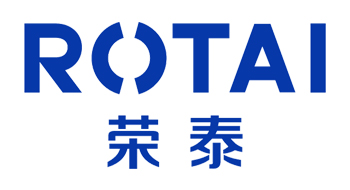+
•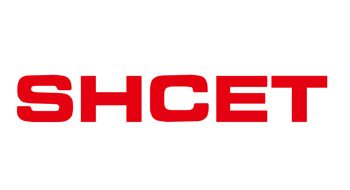+
•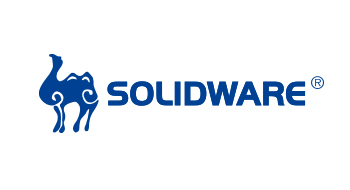+
•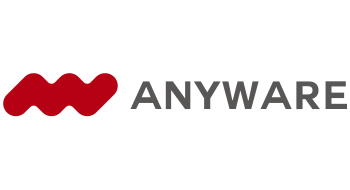+
•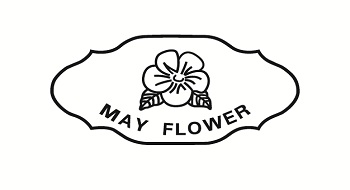+
•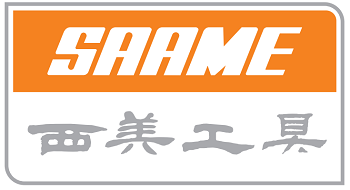+
•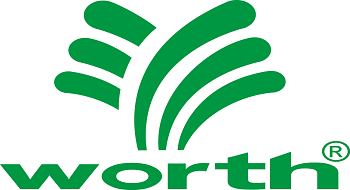+
•+
•+
•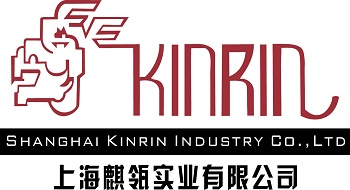+
•+
•+
•+
•+
•+
•+
•+
•+
•+
•+
•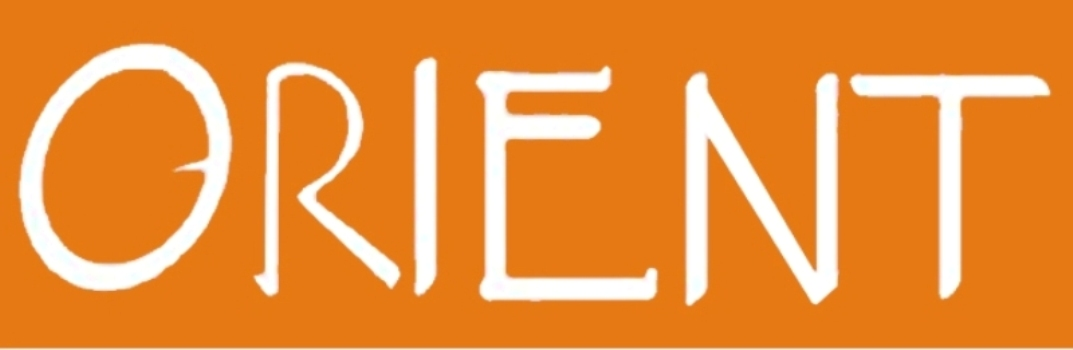+
•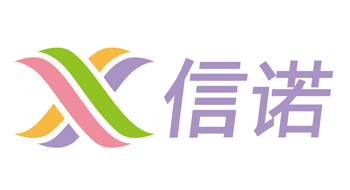+
•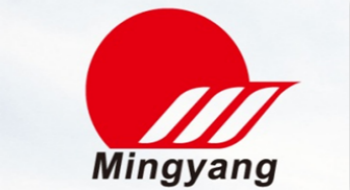+
•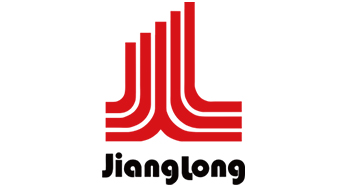+
•+
•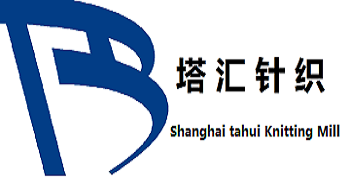+
•+
•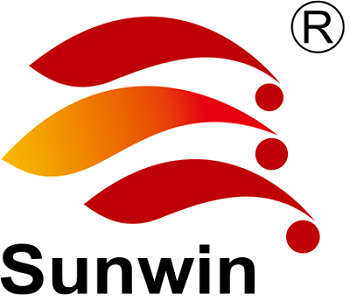+
•+
•+
•+
•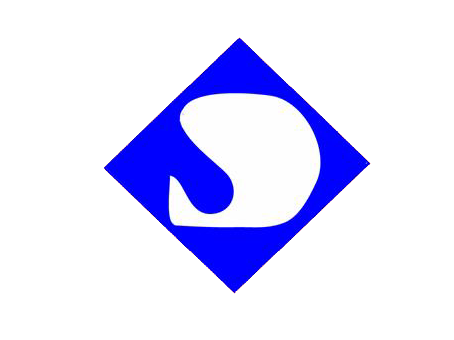+
•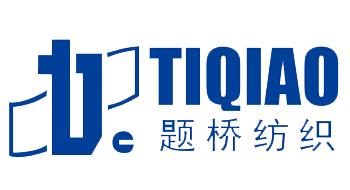+
•+
•+
•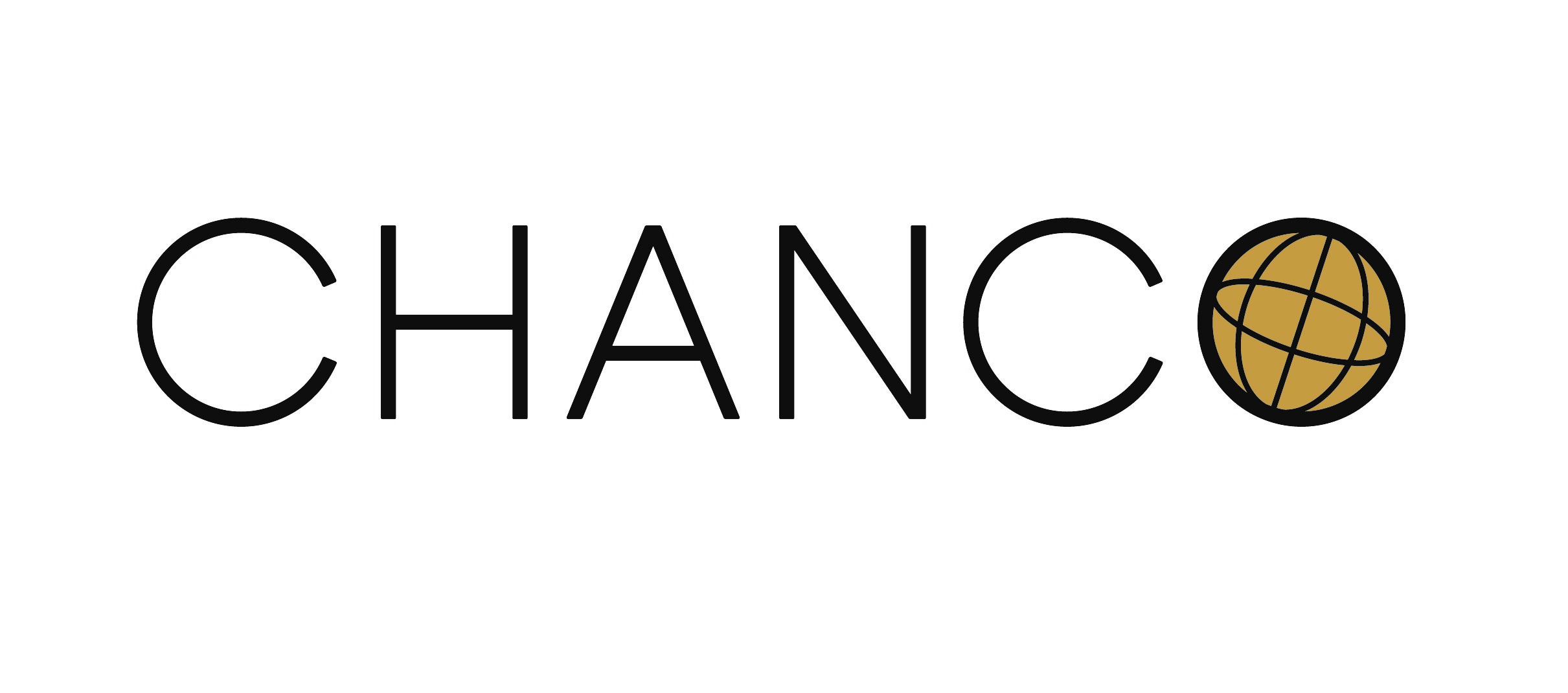+
•+
•+
•+
•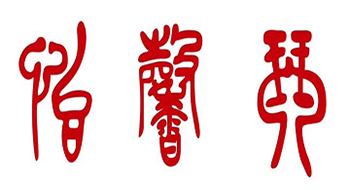+
•+
•+
•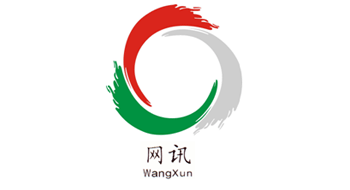+
•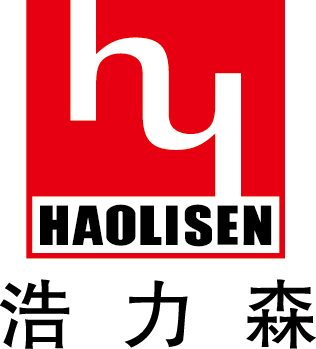+
•+
•+
•+
•+
•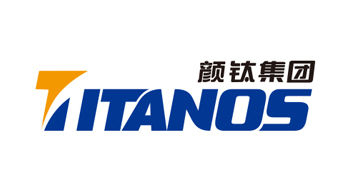+
•+

• # 5•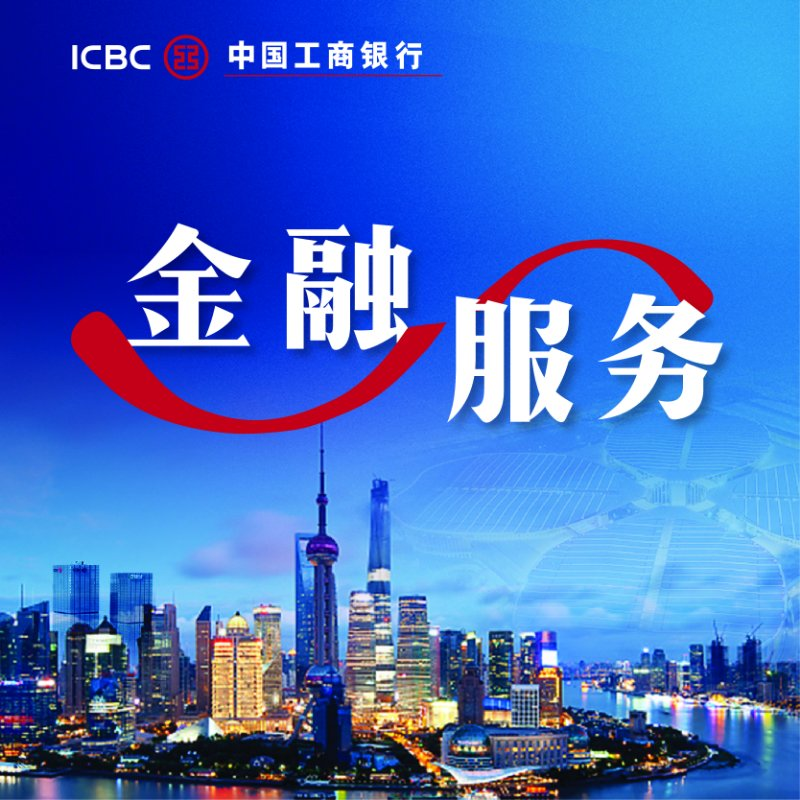•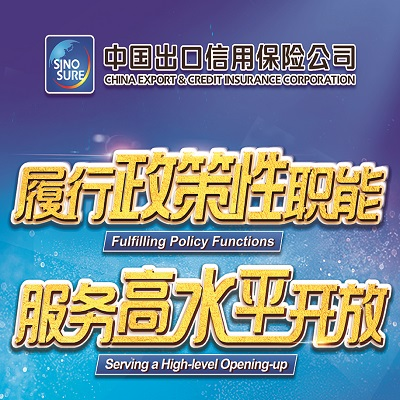•